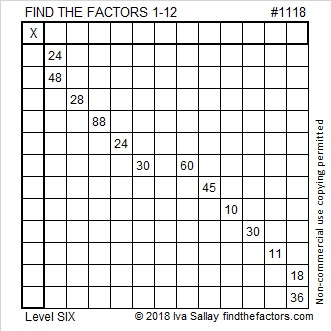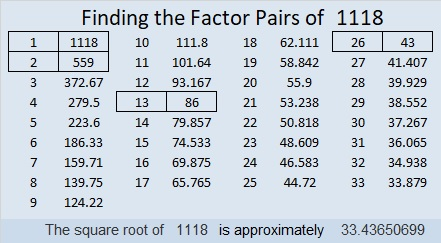# 1118 and Level 6

Each Level 6 puzzle has different logic needed to solve it. For this one, you will ask yourself over and over again, “Where is the only place ___ can go in the first column (or the top row)?” Just stick with it until all the numbers from 1 to 12 are in both of those places.Print the puzzles or type the solution in this excel file: 12 factors 1111-1119

Now here’s a little about the number 1118:

• 1118 is a composite number.
• Prime factorization: 1118 = 2 × 13 × 43
• The exponents in the prime factorization are 1, 1, and 1. Adding one to each and multiplying we get (1 + 1)(1 + 1)(1 + 1) = 2 × 2 × 2 = 8. Therefore 1118 has exactly 8 factors.
• Factors of 1118: 1, 2, 13, 26, 43, 86, 559, 1118
• Factor pairs: 1118 = 1 × 1118, 2 × 559, 13 × 86, or 26 × 43
• 1118 has no square factors that allow its square root to be simplified. √1118 ≈ 33.436511118 is the hypotenuse of a Pythagorean triple:
430-1032-1118 which is (5-12-13) times 86

1118 is also a leg in one of this year’s Pythagorean triples!
1118-1680-2018 calculated from 2(43)(13), 43² – 13², 43² + 13²

This site uses Akismet to reduce spam. Learn how your comment data is processed.